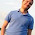## Thursday, June 30, 2011

### Bob Morris and stream algorithms

Bob Morris, one of the early contributors to UNIX and an NSA cryptographer, died on Sunday. The New York Times has a remembrance that talks about his contribution to password security and cryptography in general.

What's not mentioned in the NYT profile is that he's the Morris of the 'Morris counter'. This was arguably the very first streaming algorithm, nearly 20 years before the AMS paper and the HRR tech report first introduced streaming algorithms, five years before the Flajolet-Martin paper on counting distinct elements in a stream (also not framed as as streaming problem), and two years before the Munro-Paterson algorithm for streaming median finding.

The Morris paper is titled "Counting large numbers of events in small registers":
It is possible to use a small counter to keep approximate counts of large numbers. The resulting expected error can be rather precisely controlled. An example is given in which 8-bit counters (bytes) are used to keep track of as many as 130,000 events with a relative error which is substantially independent of the number n of events. This relative error can be expected to be 24 percent or less 95 percent of the time (i.e. σ = n/8). The techniques could be used to advantage in multichannel counting hardware or software used for the monitoring of experiments or processes.
In modern language, this can be re-rendered as:
It is possible to approximately count the number of elements in a stream using $O(\log \log n)$ bits, where $n$ is the length of the stream.
The idea is very simple. The trick is to count $C = \log n$ instead of $n$. Clearly, doing so requires only $\log\log n$ bits. Then any additive approximation to $C$ yields a multiplicative approximation to $n$.

So how do we count $\log n$ ? Let's assume for now that we're using base 2 logarithms. If the current counter value is $i$, then we "know" that the next update should come only $i$ counts later, if indeed we've seen $i$ elements. However, we don't know that the counter value reflects the true count, so we instead do it probabilistically. Specifically, toss a coin whose probability of coming up heads is $2^{-C}$, and only increment the counter if the coin comes up heads.

Phillipe Flajolet did a masterful analysis  of this approach, and showed that the expected value of $2^C$ was $n+2$, yielding an unbiased estimator for the true count. He also showed that the variance of $2^C$ is $n(n+1)/2$.

This approach yields a fixed error bound for the resulting count. The next trick is then to change the base from 2 to some parameter $a$, and then reanalyze the method. Much work later, it can be shown that with roughly $\log \log n + \log(1/\epsilon)$ bits, you can get a multiplicative approximation of $1+\epsilon$.

Postscript

Stream algorithms are a good icebreaker in an algorithms class: they're unusual, seem to solve an impossible problem, and do it really well. I also like to point out to students that three of the more important streaming algorithms were designed well before people cared about "big data" or streaming. It's a valuable lesson in the importance of understanding "old" tools.

In case you're wondering if anyone still uses Morris counters, here's a paper from IJCAI 2009 that plugs them into a larger system to maintain counts of $n$-grams when building a statistical language model.

1.Lovely description of an approach to a problem
I hadn't heard of before. Thanks, Suresh!

2.This is a great algorithm! Is there also a variant that would allow both, incrementing and decrementing, the counter?

3.Nice simple algorithm. I just wonder how much space is needed to generate the random "coin" with head-probability $2^{-C}$?

4.@Martin: there are general versions of F_1 estimation that allow for increments and decrements: they aren't as elegant as this one though.

@williampan: if I understand correctly, the log (1/eps) term relates to the space needed to generate the random coin toss from a "pure" source of randomness. There's a standard approach to doing space-efficient randomness generation due to Nisan and Wigderson that's often invoked "in theory" as a way of generating bits in small space.

5.@Suresh: I think Martin is asking about the sum of positive and negative integers, rather than F1, which is more complicated.

Does the obvious modification of the Morris counter still work? I feel like it should.